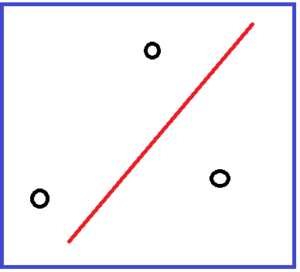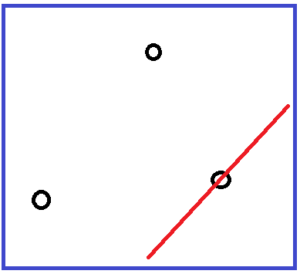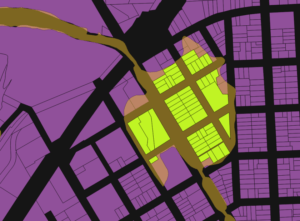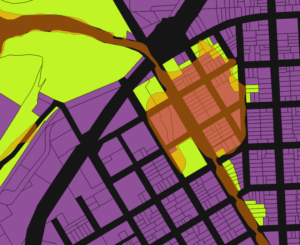# Spatial Analysis with SQL: 3-Spatial Relationship Functions

Following the two introductory articles, The spatial analysis with SQL: 1-Introduction , 2-the preconceived misconceptions , we will discuss the ten Postgis spatial relational functions (that you will also find when using Spatialite). As previously stated, this is only a small part of the available functions, but it’s really the essential core to start the spatial analysis with SQL.

## Types or dimensions of the different geometries

Before discussing the different functions we must remember what we are going to work on. The different functions have, as parameters,   two different geometries. But the geometries are not of the same type (we also refer to dimensions): we have three major types (point, line, surface) and three derived types (multipoint, multiline, multisurfaces). The distinction is important because the functions work on the determination, for each entity (geometry) of what is considered inside as well outside the entity. In the case of a layer of polygons you see right away what we are talking about and you will have no problem distinguishing the interior   polygons from their outside.

In the case of points, it starts to get tough because the inside of a point is a little harder to design. And it becomes almost metaphysical when we talk about the interior of a multipoint entity.This image can represent three point entities, in the case of a layer of points, or a single entity constituted by three points, in the case of a multipoint layer. But in both cases, the interior consists of the point or points alone. For example, in the following figure, does the line cross the geometry of the multipoint layer?The answer is NO. To traverse a given geometry, there must be a common “internal” part to both geometries.

In the following figure, however, the answer is YES, the line crosses the multipoint entity.You must understand that the interior of a line is the line itself, as for the points.

It is possible to separate the spatial relationship functions into two major groups: those that apply to all types of geometry and those that apply only to certain types.

For a detailed description of the functions, refer to the Postgis documentation: https://postgis.net/docs/manual-1.5/reference.html#Spatial_Relationships_Measurements

## Functions suitable to all types of geometry

The intersection, the difference, both of area surface or content and the touch functions accept both parameters of the function regardless the type of geometry (basic or multiple). We can therefore calculate the intersection of a multipoint layer with a polyline layer, polygons and multipolygons, etc.

### st_Intersect

This is the most generic function. It returns TRUE if the inside of a geometry occupies the same place as another inner point of the second geometry, in other words if they have at least one point of space in common. If other functions such as st_Crosses, st_Overlaps, st_touches, st_Within, or st_Contains return a TRUE value, st_Intersect will also return a TRUE value.

The following example shows the result of the query to find the plots that are affected by a flood zone of maximum risk:

SELECT plots. *
FROM plots, map_risk
WHERE ST_Intersects (plots.geometry, map_risk.geometry)
AND map_risk.zone = ‘max’Any parcel that shares even one point in the space with the flood zone is selected.

### ST_Disjoint

Is the reverse function of st_intersect. It returns TRUE if the inside of a geometry does not occupy the same place as another internal point of the second geometry, in other words if they have no point in common.

The same result is obtained with the st_intersects command with the query:
SELECT plots. *
FROM plots, map_risk
WHERE ST_Disjoint (plots.geometry, map_riskgeometry) = false
AND map_risk.zone = ‘max’

It’s a little far-fetched to make a reverse query, but if you’re really looking for disjointed geometry, it’s better to use st_Intersects = false, because a query using st_intersect is performed with spatial indexes while st_disjoint traverses sequentially all the entities, therefore response times will be significantly different!

### st_Contains and st_Within

These two functions test whether a geometry is totally inside another. st_Within (geomA, geomB) returns TRUE if the first geometry (geomA) is completely included in the other (geomB).

st_Contains (geomA, geomB) returns TRUE if the second geometry (geomB) is completely contained in the first one (geomA).

The following example shows the result of the query to find plots that are totally included in a flood zone of maximum risk:

SELECT plots. *
FROM plots, map_risk
WHERE ST_Within (plots.geometry, map_risk geometry)
AND map_risk.zone = ‘max’Only plots with all points within the flood zone are selected.

We get exactly the same result with the query

SELECT plots. *
FROM plots, map_risk
WHERE ST_Contains (map_risk.geometry, plot.geometry)
AND map_risk.zone = ‘max’

### ST_Touches

We have discussed an example of this function in the previous article. This function returns TRUE if both geometries have at least one point in common but their interiors do not intersect. In other words, only the outlines have one or more points in common.

The suitable functions according to the type of geometry

### ST_Equals

St_Equals only works on geometries of the same type. It returns TRUE if the two geometries share all the points of the space, ie if all the XY coordinates of the first geometry are identical to the coordinates of the second geometry. The order of the entities in the layer does not intervene at all in the comparison.

### ST_Crosses

St_Crosses does not apply to all combinations of geometries. It applies to the following combinations:The layers of points are excluded. The function returns TRUE if the result of the intersection is found inside the two geometries

If we go back to our previous example, the following figure shows the result of the query to find plots which are partially affected by a flood zone of maximum risk:

SELECT plots. *
FROM plots, map_risk
WHERE ST_Overlaps (plot.geometry, map_risk.geometry)
AND map_risk.zone = ‘max’Only plots with points inside AND outside the flood zone are selected.

### ST_Distance

Unlike other functions, this function does not return TRUE or FALSE but the value of the smallest calculated distance between the two geometries.

If a part (or all) of A geometry is inside the B geometry, the calculated distance will be 0.

The following example shows the result of the query to find plots within 200 meters of a flood zone of maximum risk:

SELECT plots. *
FROM plots, map_risk
WHERE ST_Distance (plots.geometry, map_risk.geometry) <200
AND map_risk.zone = ‘max’But this function is mostly used to calculate distances between geometries because it does not use spatial indexes and does a sequential processing that takes much longer. To obtain the same result as in this figure we use the function St_DWithin.

### st_DWithin

The function takes the form St_DWithin (geomA, geomB, Distance). It returns TRUE if the smallest distance between geomA and geomB is less than or equal to Distance.

For the previous example the query takes the form:

SELECT plots. *
FROM plots, map_risk
WHERE ST_Distance (plots.geometry, map_risk geometry, 200)
AND map_risk.zone = ‘max’

And we get the same map result.

This function is very useful for answering a question such as “How many plots are in a buffer of 200 meters around this stream? or that road?”,  without having to calculate the buffer. We simply test the distance between the geometries.

### st_Covers and st_CoveredBy

I left for the end these two functions which are almost equivalent to St_Contains and St_Within. The result will almost always be the same except in some very special cases. It’s a little bit twisted, but we must go back to the notion of the beginning of this article, the inside and outside of a geometry.

When we have a polygon, the inside is the space contained by the edges of the polygon. But this imaginary line that serves as an outline is not part of the interior, nor of the exterior either. It’s a limit.

When we have a line, even if it does not have a dimension   “thickness”, the line is considered to be the “inside” the geometry.

For example, if we have a regional administrative boundary, and this limit is a road that is contained in a layer of polylines, the function st_contains (polygon, line) will return FALSE because the inner area of ​​the line will not be inside the polygon (it will be on the contour).

The st_Covers function works differently. It will find that the boundary of the polygon   “covers” the stretch of road. The st_CoveredBy function tries to find out if the geometry A is covered by the geometry B and works in the same way as st_Covers.